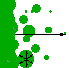12.3. Stratified Dispersal

One of the major limitations of diffusion models is the assumption of continuous spread. In nature many organisms can move or can be transferred over large distances. If spread was continuous, then islands would never be colonized by any species. Discontinuous dispersal may result in establishment of isolated colonies far away from the source population.

Passive transportation mechanisms are most important for discontinuous dispersal. They include wind-borne transfer of small organisms (especially, spores of fungi, small insects, mites); transportation of organisms on human vehicles and boats. Discontinuous long-distance dispersal usually occurs in combination with short-distance continuous dispersal. This combination of long- and short-distance dispersal mechanisms is known as stratified dispersal (Hengeveld 1989).

Stratified dispersal includes:

• establishment of new colonies far from the moving population front;
• growth of individual colonies;
• colony coalescence that contributes to the advance of population front.

 The area near the advancing population front of the pest species can be subdivided into 3 zones: Uninfested zone where pest species is generally absent Transition zone where isolated colonies become established and grow Infested zone where colonies coalesced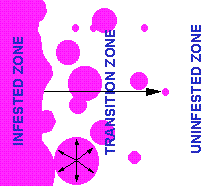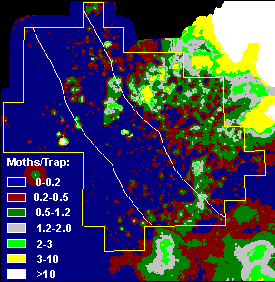A good example of a population with stratified dispersal is gypsy moth. Gypsy moth egg masses can be transported hundred miles away on human vehicles (campers, etc.). New-hatched larvae become air-borne and can be transferred to near-by forest stands. Distribution of gypsy moth counts in pheromone traps in the Appalachian Mts. (Virginia & West Virginia) in 1995 is shown in the right figure. Isolated populations are clearly visible. The US Forest Service Slow-the-Spread project has a goal to reduce the rate of gypsy moth expansion by detecting and eradicating isolated colonies located just beyond the advancing population front.

Metapopulation model of stratified dispersal

Sharov and Liebhold (1998, Ecol. Appl. 8: 1170-1179. [Get a PDF reprint!]) have developed a metapopulation model of stratified dispersal. This model is based on two functions: colony establishment rate and colony growth rate. The probability of new colony establishment, b(x), decreases with distance from the moving population front, x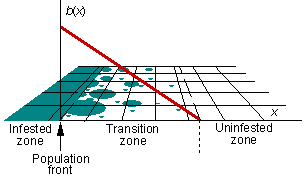Population numbers in a colony, N(a), increases exponentially with colony age, a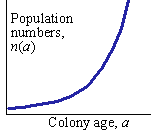n(a) = noexp(ra)

where no are initial numbers of individuals in a colony that has just established, and r is the marginal rate of population increase.

The population front is defined as the farthest point where the average density of individuals per unit area, N, reaches the carrying capacity, K:

N = K.

The rate of spread, v, can be determined using the traveling wave equation. We assume that the velocity of population spread, v, is stationary. Then, the density of colonies per unit area m(a,x) of age a at distance x from the population front is equal to colony establishment rate a time units ago. At that time, the distance from the population front was x + av. Thus, m(a,x) = b(x + av). The average numbers of individuals per unit area at distance x from the population front is equal to: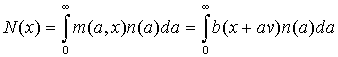where n(a) is the number of individuals in a colony of age a. The population front is defined by the condition N(0) = K. Thus, the traveling wave equation is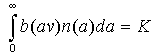This equation can be used for estimating the rate of population spread. To estimate this integral we need to define explicitly functions b(x) and n(a). We assume a linear function of the rate of colony establishment: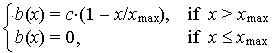The population of each colony increases exponentially

n(a) = noexp(ra)

After substituting these functions b(x) and n(a) into the traveling wave equation, we get the following equationwhere V = v/xmax is the relative rate of population spread. This equation can be solved numerically for V; and then the rate of spread is estinmated as v = V xmax.

This model can be used to predict how barrier zones (where isolated colonies are detected and eradicated) reduce the rate of population spread. We will assume that the barrier zone is placed in the transition zone at some particular distance from the population front. Because new colonies are eradicated in the barrier zone, we can set the colony establishment rate, b(x), equal to zero within the barrier zone as it is shown in the figure belowIf this new function b(x) is used with the traveling wave equation, we get the rate of spread with the barrier zone. The figure below shows the effect of barrier zone on the rate of population spread. Relative width of the barrier zone is measured as its proportion from the width of the transition zone. Relative reduction of population spread is measured as 1 minus the ratio of population spread rate with the barrier zone to the maximum rate of population spread (without barrier zone).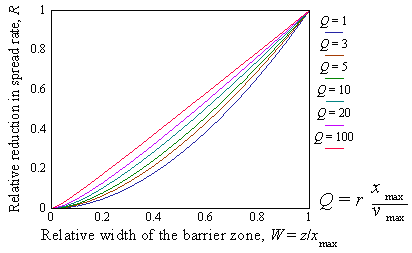This model predicted that barrier zones used in the Slow-the-Spread project should reduce the rate of gypsy moth spread by 54%. This prediction was close to the 59% reduction in the rate of gypsy moth spread in Central Appalachian Mountains observed since 1990 (when the strategy of eradicating isolated colonied has been started).

Cellular automata models of stratified dispersal

Cellular automata is a grid of cells (usually in a 2-dimensional space), in which each cell is characterized by a particular state. Dynamics of each cell is defined by transition rules which specify the future state of a cell as a function of its previous state and the state of neighboring cells. Traditional cellular automata considered close neighborhood cells only. However, in ecological applications it is convenient to consider more distant neighborhoods within specified distance from the cell.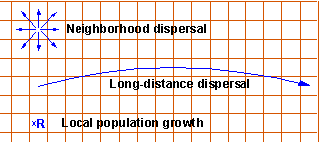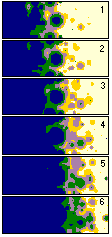The figure above shows 3 basic rules for the dynamics of cellular automata that simulates stratified dispersal: Stochastic long-distance jumps Continous local dispersal Population growth (population numbers are multiplied by R) Results of cellular automata simulation for several sequential time steps are shown at the right figure. It is seen how isolated colonies become established, grow, and then coalesce. This model was used for prediction of barrier-zone effect on the rate of population spread and the results were similar to those obtained with the metapopulation model..

References

Sharov, A. A., and A. M. Liebhold. 1998. Model of slowing the spread of gypsy moth (Lepidoptera: Lymantriidae) with a barrier zone. Ecol. Appl. 8: 1170-1179. Get a reprint! (PDF)
Sharov, A. A., and A. M. Liebhold. 1998. Bioeconomics of managing the spread of exotic pest species with barrier zones. Ecol. Appl. 8: 833-845.
Get a reprint! (PDF)
Sharov, A. A., and A. M. Liebhold. 1998. Quantitative analysis of gypsy moth spread in the Central Appalachians. Pp: 99-110. In: J. Braumgartner, P. Brandmayer and B.F.J. Manly [eds.], Population and Community Ecology for Insect Management and Conservation. Balkema, Rotterdam.
Get a reprint! (PDF)
Sharov, A. A., A. M. Liebhold and E. A. Roberts. 1998. Optimizing the use of barrier zones to slow the spread of gypsy moth (Lepidoptera: Lymantriidae) in North America. J. Econ. Entomol. 91: 165-174.
Get a reprint! (PDF)

Alexei Sharov 7/6/99